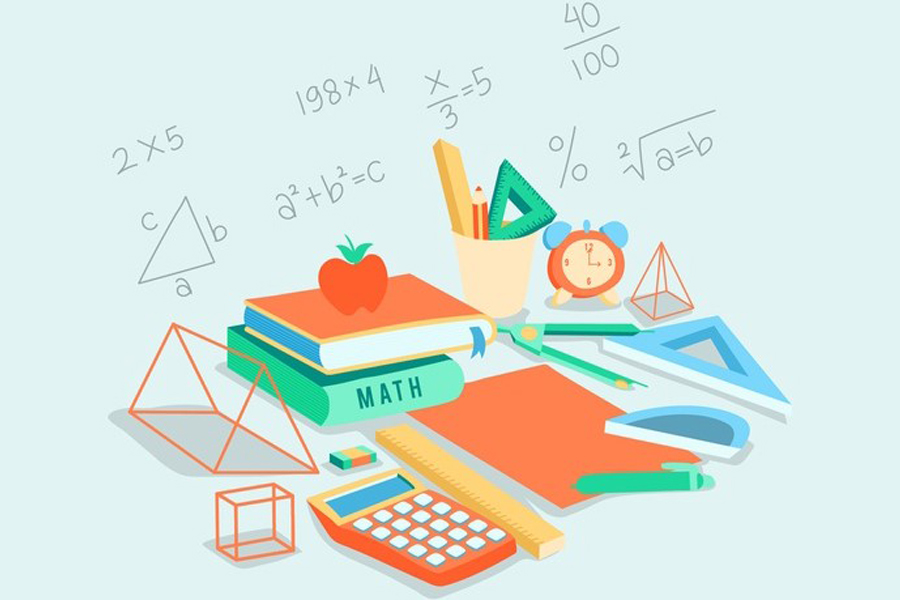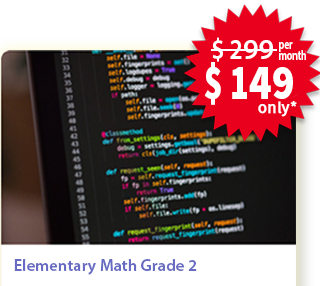## Middle School Math Grades 6 - 8[PL][11-13]## Private Lessons

• 2 sessions every week
• Each session is of 1 hour

#### Overview

Aligned with the common core curriculum of 6th to 8th grades, these sessions introduce the concepts of ratios & proportions, the number system, expressions & equations, statistics and probability, functions etc. Practicing these topics gives a solid foundation to take on the advanced high school courses like Algebra and Geometry.

#### Curriculum

###### G6-1 Ratios & Proportional Relationships - 6 2 hrs

• 1 Understand the concept of a ratio
• 2 Understand the concept of a unit rate
• 3 Use ratio and rate reasoning to solve real-world and mathematical problems

###### G6-2 The Number System - 6 4 hrs

• 4 Interpret and compute quotients of fractions
• 5 Write, read, and evaluate expressions in which letters stand for numbers.
• 6 Apply the properties of operations to generate equivalent expressions.
• 7 Greatest Common Factor and Least Common Multiplier
• 8 Positive and Negative numbers
• 9 Understand a rational number as a point on the number line
• 10 Solve real-world and mathematical problems by graphing points in all four quadrants

###### G6-3 Expressions & Equations- 6 4 hrs

• 11 Write and evaluate numerical expressions involving whole-number exponents
• 12 Apply the properties of operations to generate equivalent expressions
• 13 Identify when two expressions are equivalent
• 14 Solve one-variable equations and inequalities
• 15 Represent and analyze quantitative relationships between dependent and independent variables

###### G6-4 Geometry - 6 4 hrs

• 16 Find the area of right triangles, other triangles, special quadrilaterals, and polygons
• 17 Find the volume of a right rectangular prism with fractional edge lengths
• 18 Draw polygons in the coordinate plane given coordinates for the vertices
• 19 Three-dimensional figures using nets made up of rectangles and triangles

###### G6-5 Statistics & Probability - 6 4 hrs

• 20 Develop understanding of statistical variability
• 21 Summarize and describe distributions

###### G7-1 Ratios & Proportional Relationships - 7 4 hrs

• 22 Compute unit rates associated with ratios of fractions
• 23 Recognize and represent proportional relationships between quantities
• 24 Use proportional relationships to solve multistep ratio and percent problems

###### G7-2 The Number System - 7 4 hrs

• 25 Add and subtract rational numbers, represent addition and subtraction on a horizontal or vertical number line diagram
• 26 Multiply and divide rational numbers
• 27 Solve real-world and mathematical problems involving the four operations with rational numbers

###### G7-3 Expressions & Equations - 7 4 hrs

• 28 Use properties of operations to generate equivalent expressions
• 29 Solve multi-step real-life and mathematical problems posed with positive and negative rational numbers
• 30 Use variables to represent quantities in a real-world or mathematical problem

###### G7-4 Geometry - 7 4 hrs

• 31 Solve problems involving scale drawings of geometric figures
• 32 Draw geometric shapes with given conditions
• 33 Describe the two-dimensional figures that result from slicing three-dimensional figures
• 34 Solve real-life and mathematical problems involving angle measure, area, surface area, and volume

###### G7-5 Statistics & Probability - 7 4 hrs

• 35 Use random sampling to draw inferences about a population
• 36 Draw informal comparative inferences about two populations
• 37 Investigate chance processes and develop, use, and evaluate probability models

###### G8-1 The Number System - 8 4 hrs

• 38 Irrational numbers
• 39 Convert a decimal expansion which repeats eventually into a rational number
• 40 Use rational approximations of irrational numbers to compare the size of irrational numbers

###### G8-2 Expressions & Equations - 8 4 hrs

• 41 Work with radicals and integer exponents
• 42 Understand the connections between proportional relationships, lines, and linear equations
• 43 Analyze and solve linear equations and pairs of simultaneous linear equations

###### G8-3 Functions - 8 4 hrs

• 44 Define, evaluate, and compare functions
• 45 Use functions to model relationships between quantities

###### G8-4 Geometry - 8 4 hrs

• 46 Verify experimentally the properties of rotations, reflections, and translations
• 47 Understand congruence and similarity
• 48 Describe the effect of dilations, translations, rotations, and reflections
• 49 Use informal arguments to establish facts about the angle sum
• 50 Understand and apply the Pythagorean Theorem
• 51 Solve real-world and mathematical problems involving volume of cylinders, cones, and spheres

###### G8-5 Statistics & Probability - 8 4 hrs

• 52 Construct and interpret scatter plots for bivariate measurement data
• 53 2 Using straight lines to model relationships between two quantitative variables
• 54 2 Use the equation of a linear model to solve problems in the context of bivariate measurement data
• 55 2 Construct and interpret a two-way table summarizing data on two categorical variables

### Enroll for Math Private LessonsEnroll today to book your slot!

*Weekly 2 classes. Only limited seats available, Enroll Today.